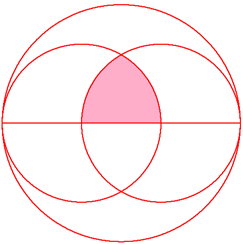# The Three Circles

Geometry Level 3In the above diagram, we have one big circle and two small circles of equal size. The straight line is the diameter of the big circle. The distance between the centers of the small circles is 1/3 the length of the diameter of the big circle.

To two decimal places, what percentage is the shaded area out of the area of the big circle?

×